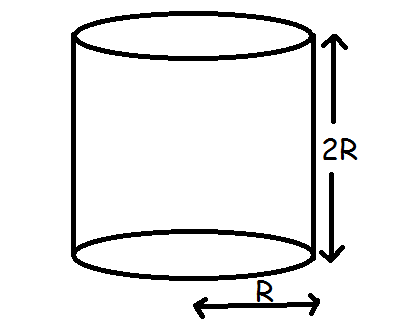# Rotating FluidA cylindrical container of dimensions shown in figure has fluid filled completely.

The container is rotated about its axis about angular velocity $\omega$.

Find the angular velocity $\omega$, such that $\dfrac14$ of the base of container is visible.

If the answer is of the form $\omega = \sqrt{\dfrac {ag}{bR} }$, where $a,b$ are coprime positive integers, find $a+b$.

×